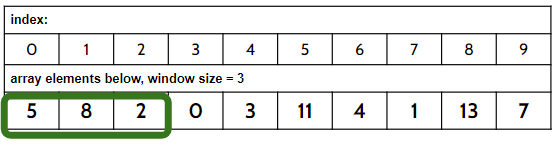# [array, window size]— Just trying to figure it all out.

After I graduated from my Flatiron, the real real challenges began: figuring out all these algorithms and data structures to help you land that perfect job(or that gets you closer to your perfect job). One such algorithm was the sliding window.

Consider this scenario: Using a given array of elements, find the largest sum within a smaller subarray of 3 consecutive numbers, and return the highest value.

Conceptually, here’s what needs to happen:
- add the first 3 numbers, log that total,
- drop the first element in our ‘window’,’ add the next element to the ‘window’
- look at the next 3 numbers, is that bigger? if so, keep the larger total.
- and on and on until we reach the last 3 numbers…
- then, return the largest sum, or indices, or first index, etc.

But. After looking at countless iterations of different codes, variable names, and wildly different approaches, I struggled to find a foothold to climb up on. It took a while, but I wanted to share the basic coding that goes along with this scenario (as I see it). I am learning, I will always welcome ways to do it better, faster, cleaner.Our array, and our first window — the next sum will be (8 + 2 + 0), and so on to the right.

Let’s start coding this out, breaking down each section as we go.
First we can define our function maxSumInArray that will take in two arguments: our array of numbers, and the size of the fixed window.
We can also create a console.log() test for our data.
We can use the table above and name it testArray.

`const maxSumInArray = (numbers, windowSize) => {}testArray = [5, 8, 2, 0, 3, 11, 4, 1, 13, 7]console.log(maxSumInArray(testArray, 3))`

We need variables for our current sum of numbers (currSum) as well as the highest total (maxSum):

`const maxSumInArray = (numbers, windowSize) => {  currSum = 0  maxSum = -1}testArray = [5, 8, 2, 0, 3, 11, 4, 1, 13, 7]console.log(maxSumInArray(testArray, 3))`

Next, we’ll create a simple “for” loop.
Then, we define currSum by adding the first number from our array.
Then the second. Then the third. Etc.
But, we want to stop at 3 total numbers.

`const maxSumInArray = (numbers, windowSize) => {  currSum = 0  maxSum = -1  for (i = 0; i < numbers.length; i++){     currSum += numbers[i]     }}testArray = [5, 8, 2, 0, 3, 11, 4, 1, 13, 7]console.log(maxSumInArray(testArray, 3))`

So, we add an “if” statement. That “if” says, once our index is 2 or greater than 2 (giving us 3 elements: numbers, numbers, numbers), stop because we need to record some totals.
Because the windowSize can fluctuate, we need to write code based on that.
“When the index is greater than or equal to (windowSize -1), do the following…”

`const maxSumInArray = (numbers, windowSize) => {  currSum = 0  maxSum = -1  for (i = 0; i < numbers.length; i++){     currSum += numbers[i]     if (i >= windowSize - 1) {     }  }}testArray = [5, 8, 2, 0, 3, 11, 4, 1, 13, 7]console.log(maxSumInArray(testArray, 3))`

On the first pass, currSum = 15 (5 + 8 + 2), and store that value in maxSum, since it’s the highest sum we have (so far).
As we cycle through, we will have to compare the maxSum value to the currSum value. And we can do that with Math.max().
Taking it a step further, since whichever value is larger needs to claim the throne as largest, we can set that equal to maxSum:

`const maxSumInArray = (numbers, windowSize) => {  currSum = 0  maxSum = -1  for (i = 0; i < numbers.length; i++){     currSum += numbers[i]     if (i >= windowSize - 1) {       maxSum = Math.max(currSum, maxSum)     }  }}testArray = [5, 8, 2, 0, 3, 11, 4, 1, 13, 7]console.log(maxSumInArray(testArray, 3))`

Check-in:
- we have a value for maxSum
- our window is looking at the first 3 elements
- our window is about to loop around and add the 4th element to our sum!
- since we only have 3 elements, we need to drop the first element:
- we must define that element by its index
- {if we’re at numbers, we want to subtract numbers from out total}
we want to subtract “2”, or one less than the windowSize…

`const maxSumInArray = (numbers, windowSize) => {  currSum = 0  maxSum = -1  for (i = 0; i < numbers.length; i++){     currSum += numbers[i]     if (i >= windowSize - 1) {       maxSum = Math.max(currSum, maxSum)       currSum -= numbers[i - (windowSize - 1)]     }  }}testArray = [5, 8, 2, 0, 3, 11, 4, 1, 13, 7]console.log(maxSumInArray(testArray, 3))`

All that’s left is :

• return the maxSum:
• handle any edge cases (array is smaller than the windowSize, windowSize = 0)
`const maxSumInArray = (numbers, windowSize) => { if (numbers.length < windowSize || windowSize < 0) return null  currSum = 0  maxSum = -1  for (i = 0; i < numbers.length; i++){     currSum += numbers[i]     if (i >= windowSize - 1) {       maxSum = Math.max(currSum, maxSum)       currSum -= numbers[i - (windowSize - 1)]     }  }  return maxSum}testArray = [5, 8, 2, 0, 3, 11, 4, 1, 13, 7]console.log(maxSumInArray(testArray, 3))`

VOILA. A fixed sliding window.

--

--